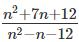Courses

# CAT Previous Year Questions: Linear Equations CAT Notes | EduRev

## Quant : CAT Previous Year Questions: Linear Equations CAT Notes | EduRev

The document CAT Previous Year Questions: Linear Equations CAT Notes | EduRev is a part of the Quant Course Quantitative Aptitude (Quant).
All you need of Quant at this link: Quant

Try yourself:What is the largest positive integer such thatis also positive integer?



Try yourself:Let A be a real number. Then the roots of the equation x2 - 4x - log2A = 0 are real and distinct if and only if



Try yourself:The quadratic equation x2 + bx + c = 0 has two roots 4a and 3a, where a is an integer. Which of the following is a possible value of b2 + c?



Offer running on EduRev: Apply code STAYHOME200 to get INR 200 off on our premium plan EduRev Infinity!

## Quantitative Aptitude (Quant)

116 videos|131 docs|131 tests

,

,

,

,

,

,

,

,

,

,

,

,

,

,

,

,

,

,

,

,

,

;